# 一．绪论

1.何谓程序设计？

程序 = 算法 + 数据结构

2.数据结构的定义

是相互之间存在一种或多种特定关系的数据元素的集合

3.数据、数据元素、数据对象的概念

数据（data）：对客观事物的符号表示，含义很广，指数、图像、声音、文本等一切计算机可以处理的事物

4.四种基本的数据结构类型

集合结构、线性结构、树型结构、图形结构

5.两种存储结构（计算机中的实现方式）

顺序存储结构、链式存储结构

6.理解顺序存储与链式存储的方式、特点、优缺点、适用情况

7.数据类型、抽象数据类型

ADT name

{                数据对象 Data

数据关系 Relation

数据操作 Operation        }

8.抽象数据类型的意义

ADT着重数据结构的操作接口，不关心具体的实现，主要是面向用户。ADT是数据结构设计所追求的目标。

9.何谓算法

算法是解决特定问题求解步骤的描述

10.算法特征

（1）有穷性：一个算法必须在执行有限步之后结束，每一步都在有穷时间内完成

（2）确定性：算法中每一步都有确切的含义，不会产生二义性

（3）可行性：算法的每一步操作都可以通过已有的可行的操作来完成

（4）输入：算法有零个或若干个数据输入

（5）输出：算法有一个或若干个数据输出

11.算法设计的要求

（1）正确性：能够解决问题

a.程序不含语法错误

b.对于几组正常的输入数据，能输出满足要求的结果

c.对于正常和异常数据，能给出满足要求的结果

d.对一切合法数据，都能够产生满足要求的结果

（2）可读性

（3）健壮性（要有异常处理机制）

（4）效率和低储存容量需求

12.算法好坏的衡量标准

（1）事后统计

（2）事前估计

a.时间复杂度估计

b.空间复杂度估计

13.时间复杂度与空间复杂度

（1）确定算法中的基本操作以及数据量的大小(由算法所涉及的局部来看)。

（2）基本操作执行情况计算出规模n的函数f(n)，并确定时间复杂度为T(n) = O(f(n))中增长最快的项/此项的系数。

# 二．线性表

1.何谓线性结构

2.线性结构主要有哪几种

线性表、栈、队列、串

3.线性表ADT，尤其是它的几个主要操作（插入元素、删除元素）

ADT List

{ 数据对象 Data={ ai , i=1,2,…n }

数据关系 （略）

数据操作：

InitList                       初始化线性表

DestroyList               销毁线性表

ClearList           清空线性表内的元素

ListEmpty         判断是否为空表

ListLength                 取得表长度

GetElement              取得某个位置的元素

LocateElement                 定位某个元素的位置

PriorElement            返回某个元素的前驱

NextElement           返回某个元素的后继

InsertElement          在某位置插入一个元素

DeleteElement                 在某位置删除一个元素

ListTraverse              遍历整个线性表

}

4.线性表的两种实现方式

顺序表、链表

5.顺序表的C语言实现

#ifndef ARRAY_LIST_H

#define ARRAY_LIST_H

#include "type.h"

array_list.c

#include <stdio.h>

#include <stdlib.h>

#include "array_list.h"

static int get_array_index_from_logic_index(int logic_index) // 从逻辑下标得到数组下标

{ return logic_index - 1;}

static int get_logic_index_from_array_index(int array_index) // 从数组下标得到逻辑下标

{return array_index + 1;}

static int is_full(const struct array_list * list) // 是否满了

{return list->capacity == list->last;}

struct array_list * array_list_init(int capacity) // 初始化

{struct array_list * tmp = (struct array_list *) malloc(sizeof (struct array_list));

if (tmp == NULL)

{perror("顺序表创建失败\n");  return NULL;}

type * data = (type *) malloc(sizeof (type) * capacity);

if (data == NULL)

{perror("顺序表创建失败\n");  return NULL; }

tmp->capacity = capacity;

tmp->data = data;

tmp->last = 0;

return tmp;}

void array_list_destroy(struct array_list * list) // 销毁

{free(list->data); free(list);}

int array_list_is_empty(const struct array_list * list) // 判空

{return list->last == 0; }

void array_list_clear(struct array_list * list) // 清空

{list->last = 0; }

int array_list_length(const struct array_list * list) // 求长度

{return list->last;}

type array_list_get(const struct array_list * list, int index) // 取得index位置的元素

{return list->data[get_array_index_from_logic_index(index)];}

void array_list_insert(struct array_list * list, int index, type data) // 在index位置添加元素

{if (is_full(list))  {perror("表是满的"); return;}

if (index < 1 || index > array_list_length(list) + 1) {perror("下表超出范围"); return; }

int array_index = get_array_index_from_logic_index(index);

int i = list->last - 1;

for (; i >= array_index; i--) {list->data[i + 1] = list->data[i];}

list->data[i + 1] = data;  list->last++;}

void array_list_insert_last(struct array_list * list, type data) // 在最后位置添加元素

{array_list_insert(list, array_list_length(list) + 1, data);}

type array_list_remove(struct array_list * list, int index) // 删除index位置的元素

{if (index < 1 || index > array_list_length(list)) {perror("index out of range"); return null_value();}

int array_index = get_array_index_from_logic_index(index);

type data = list->data[array_index];

for (int i = array_index + 1; i < list->last; i++) {list->data[i - 1] = list->data[i];}

list->last--;

return data;}

void array_list_traverse(const struct array_list * list, void (*access_function)(type data)) //遍历

{for (int i = 0; i < list->last; i++) {access_function(list->data[i]);}}

6.链表的C语言实现

单链表、循环链表、双向链表中结点的操作，比如插入、删除、查找、定位等等

7.实现的代码中，如何方便地更换数据元素的类型，以便代码复用

定义泛型泛型的定义主要有以下两种：

1.在程序编码中一些包含类型参数的类型，也就是说泛型的参数只可以代表类，不能代表个别对象。（这是当今较常见的定义）

2.在程序编码中一些包含参数的类。其参数可以代表类或对象等等。（人们大多把这称作模板）不论使用哪个定义，泛型的参数在真正使用泛型时都必须作出指明。

8.顺序表和链表的优缺点、适用场景

1.利用计算机存储的特点，实现简单，无需额外存储元素间的逻辑关系

2.随机存储

1.插入和删除操作常伴随大量的数据元素的移动

2.不利于数据规模的经常增大

a.对于预先分配空间的，无法增加空间

b.对于有自动扩充功能的，扩充之前需大 量复制已有数据元素

1.无需事先分配大块连续的内存，可根据插入删除的需要进行内存的分配和回收

2.插入和删除操作比较简单，无需进行数据的移动

链式实现的缺点

1.需要额外存储指针域

2.实现时相对比较复杂

# 三．栈与队列

1.栈的概念，ADT

（因此，对栈来说，表尾端有 其特殊含义，称为栈顶，相应地，表头端称为栈底。不含元素的空表称为空栈）

ADT Stack{ 数据元素：

略

InitStack

DestroyStack

ClearStack

StackEmpty

GetTop

Push

Pop        }

2.栈的三个基本操作

Push、Pop、GetTop

void push(struct stack * st, type data) // 进栈

{if (st->top == st->capacity)

{perror("stack is full, cannot push");return; }

st->data[st->top++] = data; }

type pop(struct stack * st) // 出栈

{if (stack_is_empty(st)) {perror("stack is empty, cannot pop");  return null_value();}

return st->data[--st->top]; }

type get_top(const struct stack * st) // 查看栈顶元素

{if (stack_is_empty(st)) {perror("stack is empty, got null");  return null_value();}

return st->data[st->top - 1]; }

3.栈的特点

4.判断能否根据某种操作顺序，得到某一个元素序列

5.栈的一些应用：括号匹配、函数调用跟踪、递归跟踪、四则运算

括号匹配思路：

1.依次读入每一个符号。

2.如果该符号是左括号（大、中、小），则入栈；

3.如果该符号是右括号，则出栈一个符号，如果与该左括号匹配，则继续；如果栈空或者取出的左括号不匹配，则判定表达式括号不匹配。

4.读完表达式，如果栈空，判定表达式括号匹配；如果栈非空，则判定表达式括号不匹配

#include<stdio.h>

#include<stdlib.h>

#include<iostream>

#include<string.h>

#define TURE 1

#define ERROR 0

#define OK TURE

using namespace std;

const int max = 10000;

char ch[max];

typedef int Status;

struct Stack  //创建栈

{       char data[max];

int top; };

Status search(char ch[], int n)

{       Stack s;

s.top = -1;

for (int i = 0; i < n; i++)

{if (ch[i] == '(' || ch[i] == '[')

s.data[++s.top] = ch[i];

if (ch[i] == ')')//左括号

{if (s.top == -1)  return ERROR;

else if (s.data[s.top] == '(')

s.top--;

else  return ERROR; }

if (ch[i] == ']') //右括号

{if (s.top == -1)  return ERROR;

else if (s.data[s.top] == '[')//匹配s.top--;

else//匹配失败return ERROR;

//处理完所有的括号查看栈是否为空

while (i == n)

{if (s.top == -1) {return OK; cout << "s.top is cleaned";}

else{return ERROR; cout << "s.top is uncleaned";}}

}}}

int main()

{       int n;//检测个数

cout << "要检测的个数:";

cin >> n;//输入n个数

int i = 1;//设置i=1

while (n--)//当n自减的时候

{cout << "第" << i << "个括号序列:";  cin >> ch;//输入括号

int len = strlen(ch);//把ch的字符长度赋值给Len

if (search(ch, len))  cout << "匹配成功" << endl;

else  cout << "匹配失败" << endl; i++;}

system("pause");

return 0;   }

1.设计算法将中序表达式转换成后序表达式

2.设计算法进行后序表达式的计算

跟踪思路：调用一次则入栈一次，

6.栈的顺序实现方式（top指针的变化方式）

#include <stdio.h>

#include <stdlib.h>

#include "stack.h"

struct stack * stack_init(int capacity)

{struct stack * st = (struct stack *) malloc(sizeof (struct stack));

if (!st) {perror("stack init failed");    return NULL; }

st->data = (type *) malloc(sizeof (type) * capacity);

if (!st->data) {perror("stack init failed");return NULL; }

st->capacity = capacity;

st->top = 0;  return st;}

7.队列的特点：FIFO

8.队列的头尾与两个基本操作

队首（front），队尾（rear），入队（en），出队（de）

void enq(struct queue * q, type data) // 入队列

{if (is_full(q)) {perror("queue is full, cannot enq");  return;  }

if (queue_is_empty(q)) { q->data[q->front] = data; }

q->data[q->rear] = data; q->rear = move_to_next(q->rear, q->length); }

type get_front(const struct queue * q) // 取得队列首元素

{if (queue_is_empty(q)) { perror("queue is empty");  return null_value(); }

return q->data[q->front];}

type deq(struct queue * q) // 出队列

{type o = get_front(q);

q->front = move_to_next(q->front, q->length);  return o; }

9．队列的顺序实现方式

front和rear的变化方式，何时表示队列满，何时表示队列空

int queue_is_empty(const struct queue * q) //判空

{return q->front == q->rear;}

static int is_full(const struct queue * q) // 判断队列满

{return move_to_next(q->rear, q->length) == q->front; }

1.串的概念（串、子串）

2.串的顺序实现方式，主要操作

ADT String{   数据对象：略

数据关系：略

基本操作：StrInit：串初始化

StrCopy：串拷贝

StrCompare：串比较

StrLength：求串长度

StrConcat：串连接

SubString：求串的某个子串

StrIndex：返回子串在串中的位置  }

3.模式匹配问题

KMP算法：该算法由Knuth，Morris，Pratt同时发现，因此命名为KMP算法，它是一种巧妙的算法，能在O(n+m)时间内完成串的模式匹配

# 五．数组与广义表

1.数组（Array） 是用来描述一组具有连续的线性关系的相同事物的概念

2.ADT Array{     数据对象：（略）

InitArray           数组初始化

DestroyArray   数组销毁

GetValue          取得某个元素

SetValue           给某个元素赋值   }

3.多维数组的定义方法

4.数组的顺序实现

5.两种保存数组的方法

1.按行存储2.按列存储

6.多维数组中基本元素的定位：

7. 二维数组元素坐标

（以行存储为例）：LOC(i, j) = LOC(0, 0) + (d2 * i + j)*L

其中d2为第二维的数组长度，L为每个基本元素所占的空间。由于有这样的计算公式，因此顺序实现的数组具有随机存取的特点。

8.矩阵的压缩存储

（1）存一半：对称矩阵（ai,j = aj,i)，上、下三角矩阵（ai,j = 0, i>j 或反过来）

（2）存坐标：稀疏矩阵（矩阵中大部分元素为0）

9.广义表

# 六．树

1.树的定义（递归方式）及表示方法

2.树的主要术语（结点、叶子结点、孩子、兄弟、双亲、结点的度、树的度、层次、深度、有序树、森林）

3.二叉树（Binary Tree）的概念（递归方式）

4.二叉树的几个性质

1.在二叉树的第i层上至多有2i-1（i≥1）个结点

2.深度为k的二叉树至多有2k-1（k≥1）个结点

3.任何一棵二叉树T，如果其终端结点数为n0，度为2的结点数为n2，则n0=n2+1

4.具有n个结点的完全二叉树的深度为（log2n）+1

5. 如果对一棵有n个结点的完全二叉树（其深度为（log2n）+1）的结点按层序编号（从第1层到第（log2n）+1）层，每层从左到右），则对任一结点i(1<i<n)，有:

(1).如果i=1，则结点i是二叉树的根，无双亲；如果i>1，则双亲是结点(i/2)

(2).如果2i>n，则结点i无做孩子（结点i为叶子结点）；否则其左孩子  是结点2i+1

(3).如果2i+1>n，则结点i无右孩子；否则其右孩子是结点2i+1

5.二叉树的链式实现，几个重要操作的实现（插入结点、删除结点）

#include "bintree.h"

#include "queue.h"

#define max(a,b) ((a)>(b)?(a):(b))

struct bintree * bintree_init()// 初始化

{struct bintree * p = (struct bintree *) malloc(sizeof (struct bintree));

if (!p) {perror("bintree init failed");  return NULL; }

p->root = NULL;  return p; }

static int is_leaf(const struct tree_node * node)//创建叶子节点

{return node->left == NULL && node->right == NULL;}

struct tree_node * insert_root(struct bintree * tree, type data) // 在空树上插入第一个结点

{if (!bintree_is_empty(tree)) { perror("tree is not empty"); return NULL; }

struct tree_node * p = create_node(data);    tree->root = p;    return p; }

static int has_left_child(const struct tree_node * node)

{return node->left != NULL; }

static int has_right_child(const struct tree_node * node)

{return node->right != NULL; }

struct tree_node * insert_left(struct tree_node * node, type data) // 插入左孩子

{if (has_left_child(node)) // 判断是否有左孩子

{       perror("node already has left child");  return NULL;   }

struct tree_node * p = create_node(data);

node->left = p;

p->parent = node;

return p; }

struct tree_node * insert_right(struct tree_node * node, type data) // 插入右孩子

{if (has_right_child(node)) // 判断是否有右孩子

{    perror("node already has right child");     return NULL;   }

struct tree_node * p = create_node(data);

node->right = p;

p->parent = node;

return p; }

struct tree_node * remove_root(struct bintree * tree) // 删除根结点

{   if (!is_leaf(tree->root)) { perror("root is not leaf");  return NULL;  }

struct tree_node * p = tree->root;

tree->root = NULL;

return p; }

static int is_left_child(const struct tree_node * child, const struct tree_node * parent)

{return child->parent == parent && parent->left == child; }

static int is_right_child(const struct tree_node * child, const struct tree_node * parent)

{return child->parent == parent && parent->right == child; }

struct tree_node * bintree_remove(struct tree_node * node) // 删除结点

{       if (!is_leaf(node)) {perror("node is not leaf");    return NULL; }

struct tree_node * parent = node->parent;

if (parent)

{   if (is_left_child(node, parent))   {parent->left = NULL; }

else if (is_right_child(node, parent))  {parent->right = NULL;}

node->parent = NULL;   }

return node;             }

6.四种二叉树遍历方法（前序、中序、后序、层次）

static void exec_pre_recurse(const struct tree_node * node, void (*access_function)(type data))

{   if (node == NULL) {  return;  }

access_function(node->data);

exec_pre_recurse(node->left, access_function);

exec_pre_recurse(node->right, access_function);   }

void pre_order_traverse(const struct bintree * tree, void (*access_function)(type data)) // 前序遍历

{ exec_pre_recurse(tree->root, access_function); }

static void exec_in_recurse(const struct tree_node * node, void (*access_function)(type data))

{  if (node == NULL) {  return;  }

exec_in_recurse(node->left, access_function);

access_function(node->data);

exec_in_recurse(node->right, access_function);   }

void  in_order_traverse(const struct bintree * tree, void (*access_function)(type data)) // 中序遍历

{exec_in_recurse(tree->root, access_function); }

static void exec_post_recurse(const struct tree_node * node, void (*access_function)(type data)) {  if (node == NULL) { return;  }

exec_post_recurse(node->left, access_function);

exec_post_recurse(node->right, access_function);

access_function(node->data);  }

// 后序遍历

void post_order_traverse(const struct bintree * tree, void (*access_function)(type data)) {

exec_post_recurse(tree->root, access_function);

}

struct bintree_queue_node // 层次遍历用到的队列，简单一点利用链表实现

{struct tree_node * data;

struct bintree_queue_node * next;  };

struct bintree_queue

{struct bintree_queue_node * front;

struct bintree_queue_node * rear;  };

static struct bintree_queue * bintree_queue_init()

{ struct bintree_queue * q = (struct bintree_queue *) malloc(sizeof (struct bintree_queue));

if (!q) { perror("bintree queue init failed"); return NULL; }

q->front = NULL;

q->rear = NULL;

return q;   }

static int bintree_queue_is_empty(const struct bintree_queue * q) // 判空

{ return q->front == NULL && q->rear == NULL; }

static void bintree_enq(struct bintree_queue * q, struct tree_node * data) // 入队列

{struct bintree_queue_node * p = (struct bintree_queue_node *) malloc(sizeof (struct bintree_queue_node));

if (!p) { perror("bintree queue init failed"); return; }

p->data = data;

p->next = NULL;

if (bintree_queue_is_empty(q))

{  q->front = p;

q->rear = p;  }

else { p->next = q->rear->next;

q->rear->next = p;

q->rear = p;  }   }

static struct tree_node * bintree_deq(struct bintree_queue * q) // 出队列

{     if (bintree_queue_is_empty(q))

{   perror("bintree queue is empty");    return NULL;    }

struct bintree_queue_node * o = q->front;

q->front = o->next;

if (q->front == NULL) {  q->rear = NULL;    }

return o->data;    }

void level_order_traverse(const struct bintree * tree, void (*access_function)(type data)) // 层次遍历

{   struct bintree_queue * q = bintree_queue_init();

bintree_enq(q, tree->root);

while (!bintree_queue_is_empty(q))

{   struct tree_node * node = bintree_deq(q);

access_function(node->data);

if (has_left_child(node)) {   bintree_enq(q, node->left);   }

if (has_right_child(node)) {    bintree_enq(q, node->right);    }      }          }

7.给定前序和中序、中序和后序的遍历序列，画出树的形状

（前中后：根的位置）

8.满二叉树与完全二叉树

9.如何把任意一棵树、森林转化为一棵等价的二叉树

10.Huffman树、如何构造

路径：树中一个结点到另一个结点间的分支

路径长度：路径中的分支数目

树的路径长度：从树根到每一个叶子结点的路径长度之和

树的带权路径长度：树中所有叶子结点的带权路径长度之和

WPL = ∑wk·lk  ， k = 1, …, n

Huffman树（最优二叉树）：设有n个权值{w1,w2,…wn}，某二叉树共有n个叶子结点，每个结点的权值分别为wi，则带权路径长度最小的二叉树称为Huffman树（最优二叉树）。

Huffman树的应用：二叉树由于只有两个分支，可以用来代表判定语句中的true和false

1.已知n个结点与各自的权值

2.以这n个结点分别作为一个只有一个根结点的树，组成集合F

3.在集合F中，寻找根结点权值最小的两棵树，构造一个新的根结点，把这两棵树分别作为该新结点的左右孩子，新的根结点的权值为两子树根结点的权值之和

4.在集合F中加入新的树，并删除原来的两棵树

5.重复第二步和第三部，直到最后只剩下一棵树，该树即为Huffman树

11.Huffman编码构造方法

前缀编码：任一字符的编码都不是另一个字符编码的前缀，如给A、B、C、D分别编码为0，00，1，01，则字符串ABCAD的编码字符串为0001001，但反过来译码时，就会产生歧义，因为该编码方式非前缀编码

设计一棵树，把各个字符看做树的叶子结点，各个字符出现的频率看做结点的权值，每个结点到其左孩子的路径为0，到右孩子的路径为1，则前缀编码的构造就是一个构造Huffman树的过程，而译码就是遍历整个Huffman树的叶子结点的过程

12.树的非递归算法

push(root)

while(栈非空)

node←pop

PreOrder:    if(not_visited(node.right))   push(node.right)

if(not_visited(node.left))

push(node.left)

if(should_visit(node))           visit(node)

else  push(node)

InOrder:           if(not_visited(node.right))     push(node.right)

if(should_visit(node))             visit(node)

else                                             push(node)

if(not_visited(node.left))        push(node.left)

PostOrder:   if(should_visit(node))              visit(node)

else                                              push(node)

if(not_visited(node.right))     push(node.right)

if(not_visited(node.left))        push(node.left)

should_visit(node)

PreOrder:  return TRUE

InOrder:  左孩子为空或左孩子已被访问过      return TRUE，否则 return FALSE

PostOrder:  左孩子为空或左孩子已被访问过 &&右孩子为空或右孩子已被访问过

return TRUE，否则  return FALSE

# 七．查找

1.查找表、关键字、主关键字、次关键字、查找、平均查找长度（ASL

查找表（Search Table）：需要被查找的数据所在的集合，通常是同一类型的数据元素（记录）构成的集合

2.静态查找表与动态查找表的区别

3.折半查找算法

折半查找（Binary Search），也叫“二分法查找”。给定一个有序的数据集，依次比较区间中间位置元素的关键字与给定值，若相等则查找成功，若不等，则把范围缩小一半，继续以上步骤，直到找到，或者区间长度小于0（未找到）。

1.设立三个指标low、high、mid，初值分别为low=0，high=N-1

2.如果low>high，则表示未找到b

3.如果low>=high，则令mid=(low+high)/2，比较b与a[mid]是否相等，如相等则表示找到该数据，如果b>a[mid]，令low=mid+1，如果b<a[mid]，令high=mid-1

4.重复2、3步骤

int binary_search(type data[], int length, type key)

{    int start = 0;

int end = length - 1;

while (start <= end)

{      int mid = (start + end) / 2;

int result = compare(data[mid], key);

if (!result)  {    return mid;      }

else if (result > 0) {     end = mid - 1;   }

else {   start = mid + 1;   }                     }

return -1;     }

4.二叉排序树（Binary Sort Tree

定义：二叉排序树或者是一棵空树，或者具有以下性质：

1、若其左子树非空，则左子树的所有结点的值均小于根结点的值；

2、若右子树非空，则右子树的所有结点的值均大于根结点的值；

3、左右子树同时也是二叉排序树

struct binary_sorted_tree * bst_init()// 初始化

{  struct binary_sorted_tree * p = (struct binary_sorted_tree *) malloc(sizeof (struct binary_sorted_tree));

if (!p) {  perror("bst init failed");  return NULL;  }

p->root = NULL;   return p;              }

static int is_leaf(const struct tree_node * node)

{    return node->left == NULL && node->right == NULL;  }

static struct tree_node * exec_clear_recurse(struct tree_node * node) {

if (node == NULL) {  return NULL;  }

node->left = exec_clear_recurse(node->left);

node->right = exec_clear_recurse(node->right);

free(node);

return NULL;     }

5.根据一串输入数据，如何构造二叉排序树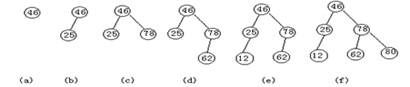6.如何在二叉排序树中进行查找

1.若根结点为空，则表示查找失败

2.若根结点非空，比较关键字与根结点的大小，若相等，则表示查找成功

3.若根结点的值小于关键字的值，则递归查找右子树，若根节点的值大于关键值，则递归查找左子树

7.二叉排序树的性能取决于什么

8.二叉平衡树的概念、平衡因子

9.二叉平衡树有什么好处

如果能构造出一棵左右子树相对“均衡”的树，则树的深度就会比较小，就能体现出树的良好性质，查找效率高。

10.四种旋转方式、各用在什么情况下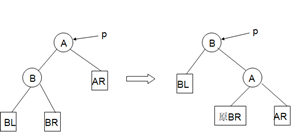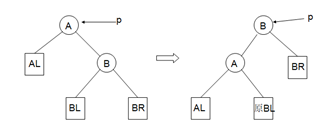：当在p的左子树根结点的右子树上插入结点导致不平衡时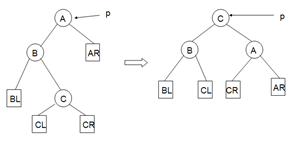：当在p的右子树根结点的左子树插入结点导致不平衡时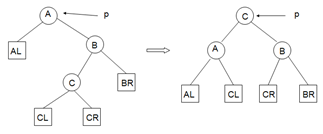11.描述下如何在二叉平衡树中插入一个新节点

1.若T为空树，则插入新元素e作为根结点

2.若T的根结点关键字等于e的关键字，则不做任何操作

3.若e的关键字小于T的根结点的关键字，则在T的左子树上递归插入e，然后检查下T的根结点的平衡因子，若平衡因子大于1或者小于-1，则根据上面四种情况之一进行调整

4.若e的关键字大于T的根结点的关键字，则在T的右子树上做类似3中的操作

12.二叉平衡树中的查找的时间复杂度

对于二叉排序树，其最大查找次数取决于树的最大深度，含有n个结点的平衡二叉树的最大深度是O(㏒2n)，因此平衡二叉排序树的性能为O(㏒2n)。

13.哈希函数

f : 关键字 → 存储位置，即，hash函数是指关键字与存储位置（哈希地址）的对应关系，只要给出关键字，就可以通过这个函数得到存储位置

14.哈希表的定义

15.几种哈希函数的构造算法

1.直接定址法：取关键字或者关键字的某个线性函数作为Hash地址，即：H(key) = key，

H(key) = a×key + b，a、b为常数

2. 数字分析法

3.平方取中法

4.折叠法

5.除留余数法（取模）：

把关键字对某个数p（不大于哈希表的表长m）取模，所得到的数作为哈希地址

H(key) = key MOD p, p≤m   （p值的选取，影响到散列之后的效果）

6. 随机数法：H(key) = random(key)，把产生的随机数做为哈希地址

16.冲突、处理冲突的基本思想

17.几个处理冲突的方法（开放地址法、再哈希法、链地址法）

1.开放定址法：处理冲突函数为Hi = (H(key) + di) MOD m, i = 1,2, …,k, k ≤ m-1，H为哈希函数，m为哈希表的表长，di为增量序列。di的选取方法：

di=1,2,3, …,m-1，称为“线性探测再散列”

di=12,-12,22,-22, …,k2,-k2, k≤m/2，称为“二次探测再散列”

di为伪随机数序列，称为“伪随机探测再散列”

2.再哈希法：Hi = RHi(key), i = 1,2, …, k， RHi为不同的一些哈希函数

3.链地址法：将所有冲突的记录存储在一个线性链表中，哈希函数得到的地址中保存这个链表

18.哈希表中查找一个元素的过程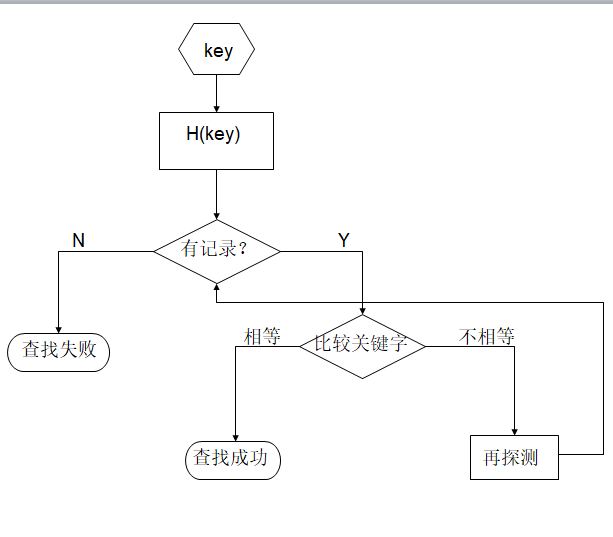1.哈希函数

哈希函数的好坏，影响出现冲突的频繁程度。一个均匀的哈希函数，对一组关键字，产生的冲突可能性都相同，它不是影响ASL的决定性因素

2.冲突处理方法

针对所介绍的几个冲突处理方法（线性探测、二次探测、随机探测、再探测、链地址），各自的ASL不同

3.装填因子（衡量哈希表的装满程度）影响该哈希表的ASL

α（装填因子）= 表中记录数/哈希表长度

# 八．排序

1.排序的基本概念、稳定、内部排序与外部排序

2.简单排序方法有：插入排序、冒泡排序、选择排序

3.先进排序方法有：shell排序、快速排序、堆排序、归并排序

4.基数排序

5.Shell排序中的步长序列（或增量因子）、shell排序的大致过程，shell排序快慢的关键

6.快速排序中：支点（pivot），算法的基本过程，快慢取决于什么

7.会使用C库中自带的快速排序函数qsort

8.什么叫做堆（注意与排序树的差别）、最大堆、最小堆

9.描述堆排序的过程（两个主要过程），每一次建堆之后会形成什么状态

10.何为归并，归并排序的主要过程

11.多关键字排序

12.MSD方法与LSD方法

13.基数排序的过程（详细描述）（分配与收集）

14.各种排序方法的比较（最坏、平均时间复杂度、所需辅助空间、稳定与否）

01-04394210-011万+
12-081340
08-10647
11-047564
03-151204
02-1390
06-04398
07-17358
12-15702
05-09240
06-232634
11-061万+
09-295804
03-011837
10-084293
©️2020 CSDN 皮肤主题: 书香水墨 设计师:CSDN官方博客